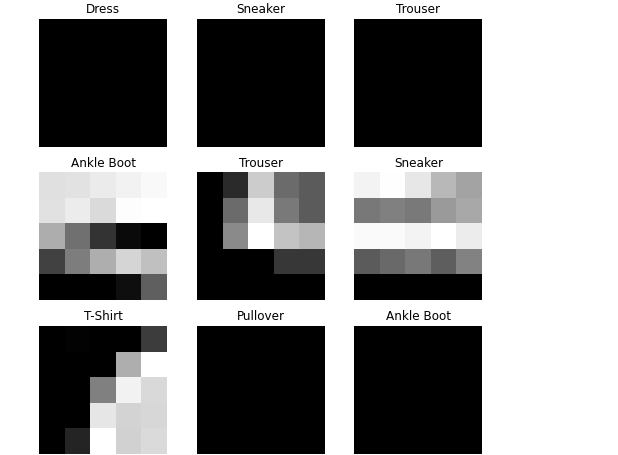# Data Augmentation Fashion MNIST Image

Hi i need to Augment Fashion MNIST with vertical flip and random crop upto 5 pixels in x and y
I used the following commands for training and test data for transform
transform=transforms.Compose(
[transforms.ToTensor(),
transforms.RandomVerticalFlip(p=0.5),
])

and i get this result.I think i am not doing it right.Any suggestions on how to improve it?In this case you probably want `RandomResizedCrop` instead: torchvision.transforms — Torchvision 0.11.0 documentation

Assuming your source images are 28x28, 5 pixels or 23x23 means a minimum scale of ~0.675. Could you check the output with `transforms.RandomResizedCrop(input_size, scale=(0.675, 1.0))` is what you’re looking for?

How do we check the images are 28 x28?

hmmm now the output is like this .I used inputsize as 23.It seems way better.How did you decide the scale values to be 0.675 to 1?

How do we check the images are 28 x28?

You should be able to do this by printing `.shape` on the input tensor without any transformation (e.g., `transform=transforms.Compose( [transforms.ToTensor(),]`).

How did you decide the scale values to be 0.675 to 1?

This was a guess based on your description of cropping 5 pixels e.g., 23x23/28x28 is approximately 0.675, but this is ultimately a tunable hyperparameter and you can adjust this (e.g., based on validation accuracy).

1 Like

Thank you so much for clarifying

If we were to use a normal randomcrop and pad,Is there still a possible way to do it?

This isn’t very common so I’m not sure what the cleanest way to do this is without writing a custom transformation.
What does `transforms.RandomCrop(23)` give you? or `transforms.RandomCrop(28, padding=2)`? Note that I picked 2 in this case because I’m not sure if you meant 5 pixels long each axis or each border.